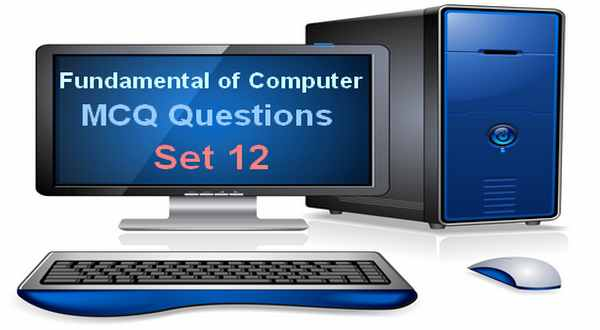# Fundamentals of Computer MCQ Questions With Answers Set 12Computer mcqs, computer mcqs with answers, computer mcq book, fundamentals of computer mcqs questions with answers set 12. This mcqs set lists the mcqs of the basics of computer. A computer is an electronic machine which takes user input in the form of data and process it with the help of their brain called central processing unit and produced the useful information either in soft form or in hard copy. A personal computer have input unit, output unit, central processing unit and memory unit. The input unit consist of the widely used input devices such as keyboard, mouse, joystick etc. Output unit consist of monitor, printer, plotter etc. Central processing unit consist of the arithmetic and logic unit and control unit. The memory unit have two types either primary or secondary. In computer ram act as primary memory while hard disk act as secondary memory.

This fundamentals of computer mcqs set includes the computer mcqs questions such as memory of the computer, computer languages, generation and invention of the computer, the functions of the earlier computers, computer used technologies inventors, measuring units of computer memory, the usage of the earlier developed computer languages, computer errors, abbreviations of computer terms and some questions related to printers. All these mcqs are grabbed from the previous jobs and examination tests and can be helpful to prepare your jobs or admission tests.

## Fundamentals of Computer MCQ Questions With Answers Set 12

1. Which of the following require large computers memory ?
A). Imaging
B). Graphics
C). Voice
D). All of Above

2. Which of the following is machine independence program ?
A). High level language
B). Low level language
C). Assembly language
D). Machine language

3. When was the first electro-mechanical computer developed ?
A). 1880
B). 1990
C). 1944
D). None of above

4. The first machine to successfully perform a long series of arithmetic and logical operations was ?
A). ENIAC
B). Mark-I
C). Analytic Engine
D). UNIVAC-1

5. Who invented Integrated Circuits?
A). Jack Kilby
B). Robert Noyce
C). Both of above
D). None of above

6. Which one is the largest space?
A). Kilobyte
B). Petabyte
C). Terabyte
D). Gigabyte

7. FORTRAN programming language is more suitable for ____ ?
B). Marketing Applications
C). Scientific Applications
D). None of the above

8. The brain of any computer system is ?
A). Control Unit
B). Arithmetic Logic Unit
C). Central Processing Unit
D). Storage Unit

9. Analog computer works on the supply of ?
A). Continuous electrical pulses
B). Electrical pulses but not continuous
C). Magnetic strength
D). None of the above

10. An error in software or hardware is called a bug. What is the alternative computer jargon for it?
A). Leech
B). Squid
C). Slug
D). Glitch

11. What does DMA stand for?
A). Distinct Memory Access
B). Direct Memory Access
C). Direct Module Access
D). Direct Memory Allocation

12. The advantage of COM are its __ and __ ?
B). Compact size, speed

13. In a computer _____ is capable to store single binary bit ?
A). Capacitor
B). Flip flop
C). Register
D). Inductor

14. The BIOS is the abbreviation of ________. ?
A). Basic Input Output System
B). Best Input Output System
C). Basic Input Output Symbol
D). Base Input Output System

15. Which printer is very commonly used for desktop publishing ?
A). Laser printer
B). Inkjet printer
C). Daisywheel printer
D). Dot matrix printer

16. IBM 1401 is ?
A). First Generation Computer
B). Second Generation Computer
C). Third Generation Computer
D). Fourth Generation Computer

17. Most of the first generation computers were ?
A). Special purpose computers
B). General purpose computers
C). Both of above
D). None of above

18. Floppy disks typically in diameter ?
A). 3
B). 5.25
C). 8
D). All of above

19. The output quality of a printer is measured by ?
A). Dot per inch
B). Dot per sq. inch
C). Dots printed per unit time
D). All of above

20. On a PC, how much memory is available to application software?
A). 1024 KB
B). 760 KB
C). 640 KB
D). 560 KB